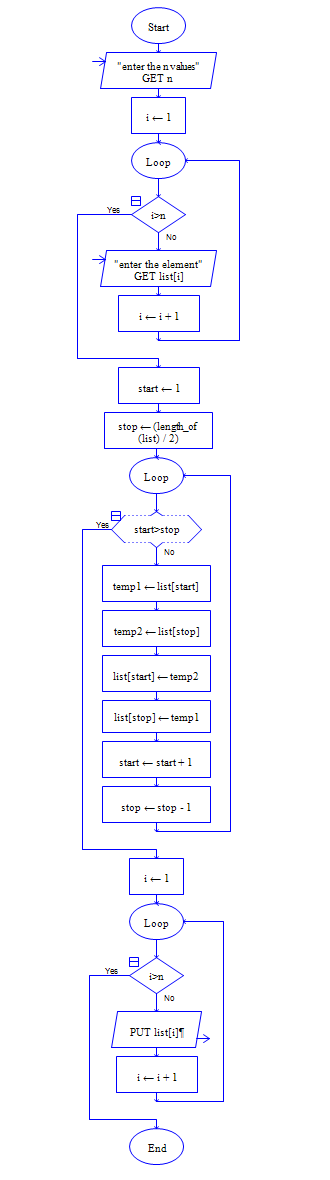# Java Program to Reverse the Elements of Array

Get array size n and n elements of array, then reverse the n elements.

Sample Input 1:

5

5 7 9 3 1

Sample Output 1:

1 3 9 7 5

#### Flow Chart Design#### Program or Solution

``` //To Reverse The Elements In Array... import java.util.*; class Program { public static void main(String args[]) { int size,i,j; Scanner sc=new Scanner(System.in); System.out.println("Enter The Size Of The Array:"); size=sc.nextInt(); int a[]=new int[size]; System.out.println("Enter The Array Elements:"); for(i=0;i<size;i++) { a[i]=sc.nextInt(); } for(i=0,j=size-1;i<j;i++,j--) { int temp=a[i]; a[i]=a[j]; a[j]=temp; } System.out.println("The Output Is:"); for(i=0;i<size;i++) { System.out.println(" "+a[i]); } } } ```